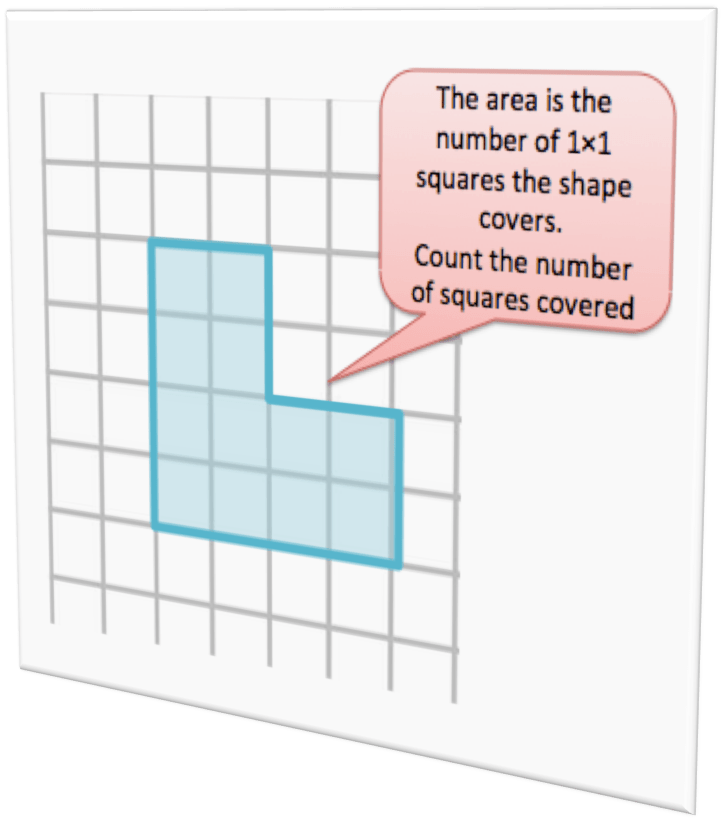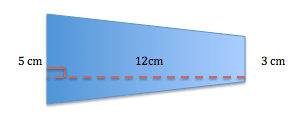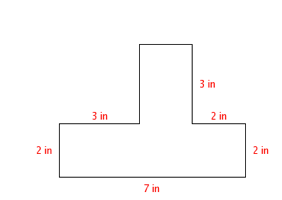# Area of Triangles and Other Polygons

Recall that the area of a parallelogram is base x height.

If you divide a parallelogram into two congruent triangles, the area of each triangle is ½ x base x height.So the Area of a Triangle: A = ½ bh

In order to use the lengths of the legs of the triangle as the base and height, the legs must meet at a 90o angle.

## Area of a Trapezoid

A trapezoid can be divided into a rectangle and two triangles.   The area of a trapezoid is the sum of the areas of the rectangle and the triangles.So the Area of a Trapezoid is## Steps

You can follow these steps at this site. Go there to read these steps and see the images.

Identify the length of both bases. The bases are the two parallel sides of the trapezoid. Let’s call them sides a and b. Side a is 8 cm long and side b is 13 cm long.
Add the lengths of the bases. Add 8 cm and 13 cm. 8 cm + 13 cm.
Identify the height of the trapezoid. The height of the trapezoid is perpendicular to the bases. In this example, the height of the trapezoid is 7 cm.
Multiply the sum of the lengths of the bases by the height. The sum of the lengths of the bases is 21 cm and the height is 7 cm. 21 cm x 7 cm = 147 cm2.
Divide the result by two. Divide 147 cm2 by 2 to get the final answer. 147 cm2/2 = 73.5 cm2. The area of the trapezoid is 73.5. You have just followed the formula for finding the area of a trapezoid, which is [(b1 + b2) x h]/2.

## Area of Irregular PolygonsYou can find the area of irregular polygons by decomposing the polygon into rectangles, triangles and other shapes.

## Practice

Find the area of each shape.This is 27, 24, 18, 30.(sources – GVLWikihow)